# A-Level物理考试真题长什么样，一起来看看!

A-Level物理考试的内容涵盖的范围比较广，其中包括普通物理(general physics)、牛顿力学(newtonian mechanics)、物质(matter)、 振动和波(oscillations and waves)、电和磁(electricity and magnetism)、现代物理(modern physics)、应用物理(applications of physics)几个大的板块。A-Level物理涉及到的知识面要大于国内高中物理课程，甚至还包括了一部分高校的物理知识。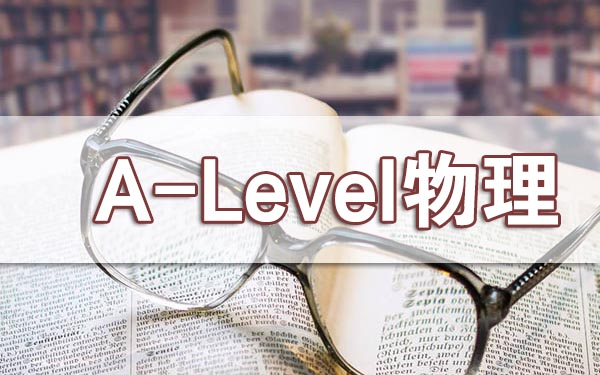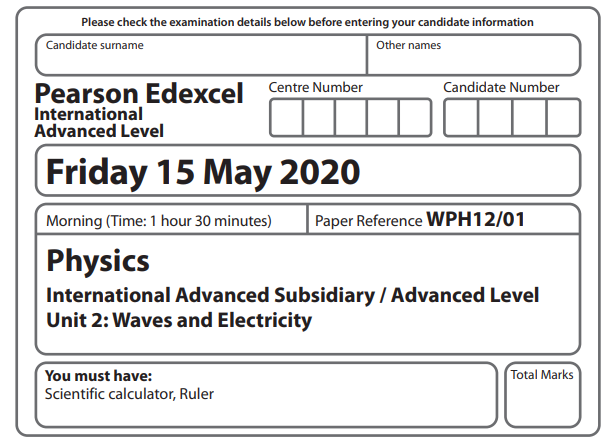Instructions

• Use black ink or ball‑point pen.

• Fill in the boxes at the top of this page with your name, centre number and candidate number.

• Answer all questions. • Answer the questions in the spaces provided – there may be more space than you need.

• Show all your working out in calculations and include units where appropriate.

Information

• The total mark for this paper is 80.

• The marks for each question are shown in brackets – use this as a guide as to how much time to spend on each question.

• In questions marked with an asterisk (*), marks will be awarded for your ability to structure your answer logically showing how the points that you make are related or follow on from each other where appropriate.

• The list of data, formulae and relationships is printed at the end of this booklet.

• Try to answer every question.

SECTION A

For questions 1–10 select one answer from A to D and put a cross in the box .

If you change your mind, put a line through the box and then mark your new answer with a cross .

1.Waves can be represented on graphs of displacement against distance and displacement against time.

Which of the following properties can only be determined by combining information from both graphs?

A amplitude

B frequency

C speed

D wavelength

2.Two waves from the same source arrive at a point with a path difference of 3 8 λ , where λ is the wavelength.

Which of the following is the phase difference of the waves at this point?

A 34°

B 68°

C 135°

D 270°

3.A diffraction grating has 300 lines per mm. When laser light is directed towards the diffraction grating, a diffraction pattern is observed on a screen. The position of a first order maximum on the screen is shown in the diagram.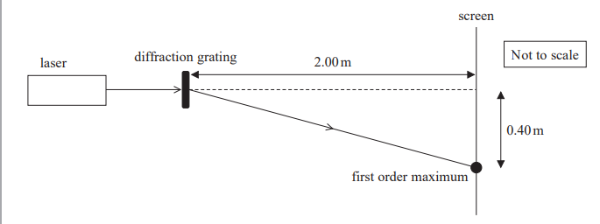Which of the following calculations should be used to determine the wavelength, in mm, of the laser light used?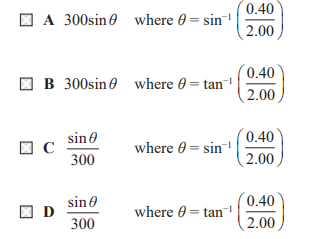4.Which of the following statements about physical quantities or units is correct?

A Current is a derived quantity.

B Power is a derived quantity.

C The coulomb is a base unit.

D The volt is a base unit

5.Ultrasound has a number of different uses in medicine.

Which of the following is the main reason why ultrasound is not used to perform scans of the chest and lungs?

A The air in the lungs causes most of the ultrasound to be reflected back.

B The duration of the pulses of ultrasound limits the amount of detail that can be seen.

C The wavelength of the ultrasound is too long.

D Ultrasound can damage the lungs.

6.Which electrical component has the current-potential difference graph shown?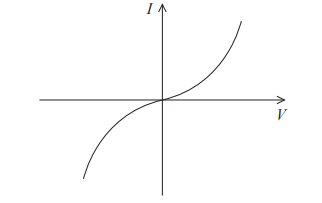A diode

B filament lamp

C resistor

D thermistor

7.A small metal cube, with sides of length x, is connected in a circuit. The current through the cube, between opposite faces, is I and the potential difference across the cube is V.

Which of the following gives the resistivity of the metal?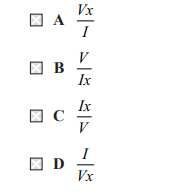8.Which of the following statements about waves is correct?

A Both longitudinal and transverse waves can be polarised.

B Neither longitudinal nor transverse waves can be polarised.

C Only longitudinal waves can be polarised.

D Only transverse waves can be polarised.

9.Two copper wires are placed in series in a complete circuit. The electrons in the two wires have different values of drift velocity.

Which of the following must be different for the two wires?

A charge carrier density

B cross-sectional area

C current

D length

10.The circuit shows a potential divider, including a battery of negligible internal resistance and a light dependent resistor (LDR). The potential difference (p.d.) across the fixed resistor is measured using a voltmeter.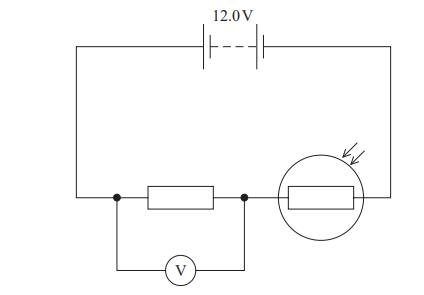A If the resistance of the LDR halves, the voltmeter reading halves.

B Increasing the light intensity would decrease the voltmeter reading.

C Increasing the light intensity would have no effect on the voltmeter reading.

D The voltmeter reading added to the p.d. across the LDR will always be 12.0 V.

TOTAL FOR SECTION A = 10 MARKS

## 推荐阅读

【责任编辑】：成都新航道小编 版权所有：转载请注明出处

## SAT资料•官方客服微信